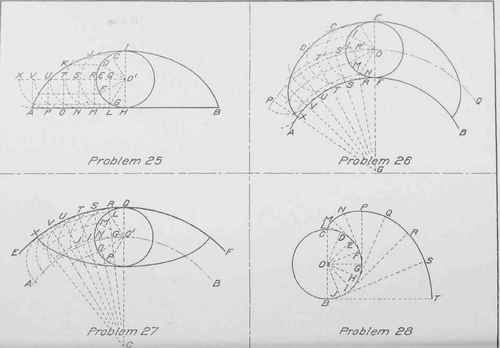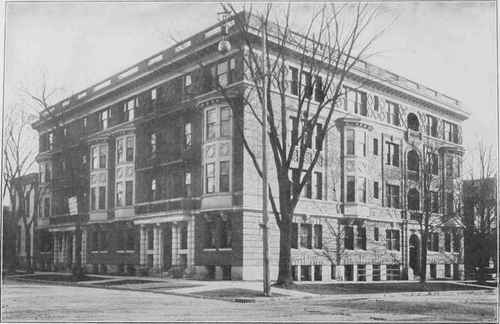PLATE VIIIMARLBORO FLAT BUILDING, MILWAUKEE, WIS.

Fernekes & Cramer, Architects, Milwaukee, Wis.

Walls of Continental Paving Brick; Entrances and all Stonework of Bedford Stone; Cornice of Galvanized Iron; Balconies of Wrought Iron. Composition Roof. All Public Halls and All Living Rooms of Oak Finish; Bedrooms, Poplar, for Enamel;SECOND-STORY PLAN OF MARLBORO FLAT BUILDING, MILWAUKEE, WIS.

Fernekes & Cramer, Architects, Milwaukee, Wis. Exterior View Shown on Opposite Page.

Draw E F about 3 inches long and mark the point X, 1 inch from E and the point Y, 1 inch from X. With the triangle and T-square, draw the rectangles A B D C and O P Q R such that A B is 1 inch in length and A C, 3 inches in length. Divide A E and A B into the same number of equal parts. Connect Y with the points T, U, and V, on A E, and connect X with L, M, and N, on A B. The first point on the curve is at A; the next is at the intersection of T Y and L X; the third is at the intersection of U Y and M X. The remaining points are found in the same manner. Repeat the process for X C and the right-hand curve P Y Q.

Inking. In inking the figures on this plate, use the French or irregular curve and make full lines for the curves and their axes. Dot the construction lines as usual. Ink in all the construction lines used in finding one-half of a curve, and in Problems 19, 20, 23, and 24 leave all construction lines in pencil except those inked. In Problems 21 and 22 erase all construction lines not inked. The trammel used in Problem 21 may be drawn in the position shown, or outside of the ellipse in any convenient place.

The same lettering should be done on this plate as on previous plates

## Plate VIII

Penciling. In laying out Plate VIII, draw the border lines and horizontal and vertical center lines as in previous plates, dividing the plate into four spaces.

Problem 25. To construct a cycloid when the diameter of the generating circle is given.

With O' as a center and a radius of 7/8 inch draw a circle, and, tangent to it, draw the indefinite horizontal straight line A B. Divide the circle into any number of equal parts - 12 for instance - and through these points of division C, D, E, F, etc., draw horizontal lines. Now with the dividers set so that the distance between the points is equal to the chord of the arc C D, mark off the points L, M, N, O, P, on the line A B, commencing at the point II. At these points erect perpendiculars to the center line G O' which is the line of centers of the generating circle as it rolls along the line A B. With the intersections Q, R, S, T, etc., as centers describe arcs of circles as shown. The points on the cycloid will be the intersections of these arcs and the horizontal lines drawn through the points C, D, E, F, etc. Thus the intersection of the are whose center is Q and the horizontal line through C is a point I on the curve. Similarly, the intersection of the arc whose center is R and the horizontal line through D is the point J on the curve. The remaining points on the left, as well as those on the right, are found in the same manner. To obtain great accuracy in this curve, the circle should be divided into a large number of equal parts, because the greater the number of divisions the less the error due to the difference in length between a chord and its arc.

Problem 26. To construct an epicycloid when the diameter of the rating circle and the diameter of the director circle are given. The epicycloid and the hypocycloid may be drawn in the same manner as the cycloid if arcs of circles are used in place of the horizontal lines. With 0 as a center and a radius of 3/4 inch describe a circle. Draw the diameter E F of this circle and produce E F to G such that the line F G is 2 3/4 inches long. With G as a center and a radius F G, describe the arc A B of the director circle. With the same center G, draw the arc P Q which will be the path of the center of the generating circle as it rolls along the arc A B. Now divide the generating circle into any number of equal parts - twelve for instance - and through the points of division H, I, L, M, and N, draw arcs having G as a center. With the dividers set so that the distance between the points is equal to the chord H I, mark off distances on the director circle A F B. Through these points of division R, S, T, U, etc., draw radii intersecting the arc P Q in the points R', S', T', etc., and with these points as centers describe arcs of circles as in Problem 25. The intersections of these arcs with the arcs already drawn through the points H, I, L, M, etc., are points on the epicycloid. Thus the intersection of the circle whose center is R' with the are drawn through the point H is a point upon the curve. Also the arc whose center is S' with the arc drawn through the point I is another point on the curve. The remaining points are found by repeating this process.

Problem 27. To draw an hypocycloid when the diameter of the generating circle and the radius of the director circle are given.

With 0 as a center and a radius of 4 inches describe the arc E F, which is the arc of the director circle. Now with the same center and a radius of 3 1/4 inches, describe the are A B, which is the line of centers of the generating circle as it rolls on the director circle. With 0' as a center and a radius of 3/4 inch describe the generating circle. As before, divide the generating circle into any number of equal parts - 12, for instance - and with these points of division L, M, N, 0, etc., draw arcs having C as a center. Upon the arc E F, lay off distances Q R, R S, S T, etc., equal to the chord Q L. Draw radii from the points R, S, T, etc., to the center of the director circle 0 and describe arcs of circles having a radius equal to the radius of the generating circle, using the points G, I, J, etc., as centers. As in Problem 26, the intersections of the arcs are the points on the hypocycloid. By repeating this process, the right-hand portion of the curve may be drawn.

Problem 28. To draw the involute of a circle when the diameter of the base circle is known.

With the point 0 as a center and a radius of 1 inch, describe the base circle. Divide the circle into any number of equal parts - 16, for instance - and draw radii to the points of division. At the point D, draw a light pencil line perpendicular to 0 D. This line will be tangent to the circle. Similarly at the points E, F, G, H, etc., draw tangents to the circle. Set the dividers so that the distance between the points will be equal to the chord of the arc C D, and measure this distance from D along the tangent. From the point E, measure on the tangent a distance equal to two of these chords; from the point F, three divisions; and from the point G, four divisions. Similarly, measure distances on the remaining tangents, each time adding the length of the chord. This will give the points L, M, N, P, etc., to T. The curve drawn through these points will be the involute of the circle.

Inking. Observe the same rules in inking Plate VIII as were given for Plate VII. In Problems 25 and 26 the arcs and lines used in locating the points of the other half of the curve may be left in pencil. In Problem 28, all construction lines should be inked. After completing the problems the same lettering should be done on this plate as on previous plates.PRAIRIE FARM BUNGALOW IN A WESTERN STATE R. C. Spencer, Jr., Architect, Chicago, 111. The Windmill Tower Contains the Staircase Leading to Attic and Cellar.PLAN OF PRAIRIE FARM BUNGALOW.

R. C. Spencer, Jr., Architect, Chicago, 111.

Credit is Due " The House Beautiful" Magazine, Owner of the Copyright, for the Use of this Picture.